# Plus Two Physics Chapter Wise Questions and Answers Chapter 1 Electric Charges and Fields

Students can Download Chapter 1 Electric Charges and Fields Questions and Answers, Plus Two Physics Chapter Wise Questions and Answers helps you to revise the complete Kerala State Syllabus and score more marks in your examinations.

## Kerala Plus Two Physics Chapter Wise Questions and Answers Chapter 1 Electric Charges and Fields

### Plus Two Physics Electric Charges and Fields NCERT Text Book Questions and Answers

Question 1.
What is the force between two small charged spheres having charges of 2 × 10-7C and 3 × 10-7 C placed 30cm apart in air?
Given q1 = 2 × 10-7C, q1 = 3 × 10-7 C
r = 30cm = 0.3m
Force of repulsion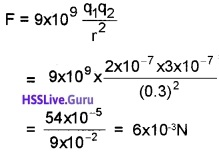Question 2.
When a glass rod is rubbed with a silk cloth, charges appear on both. A similar phenemenon is observed with many other pairs of bodies. Explain how this observation is consistent with the law of conservation of charge.
When a glass rod is rubbed with silk, the charges developed on the glass rod and the piece of silk are equal and opposite. Similar is the case in other pair of bodies. So electric charge is neither be produced nor destroyed but simply transferred from one body to another, hence is consistent with the law of conservation of charge.

Question 3.
An electric dipole with dipole moment 4 × 109 cm is aligned at 30° with the direction of a uniform electric field of magnitude 5 × 104NC-1. Calculate the magnitude of the torque acting on the dipole.
Given
p = 4 × 10-9cm, θ = 30°, E = 5 × 104 NC-1, τ = ?
Since τ = pEsinθ
= 4 × 109 × 5 × 104 × sin 30°
= 20 × 10-5 × 1/2 = 10-4Nm.Question 4.
A Polythene piece rubbed with wool is found to have a negative charge of 3 × 107C.

1. Estimate the number of electrons transferred from which to which?
2. Is there a transfer of mass from wool to polythene?

1. Charge on one electron
∴ Number of electrons in the given charge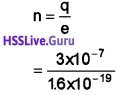= 1.875 × 1012 = 2 × 1012

2. Since woold gets negative charge on rubbing with polythene, wool must gain electrons from polythene.
∴ Ideally speaking there must be a transfer of mass due to transfer of electrons but since the mass of electron is very small, this transfer of mass may be negligible (=2 × 1018kg).

Question 5.
A uniformly charged conducting spheres of 2.4m diameter has a surface change density of 80.0m cm-2.

1. Find the charge on the sphere.
2. What is the total electric flux leaving the surface of the sphere?

Given σ = 80.0µcm-2 = 80 × 10-6cm-2
D = 2.4m, r=1.2m
1. Charge on the sphere,
q = σ × 4πr2
= 80 × 10-6 × 4 × 3.142 ×(1.2)2
= 1.45 × 10-3C.

2. Electric flux,
Φ = $$\frac{q}{\varepsilon_{0}}=\frac{1.45 \times 10^{-3}}{8.854 \times 10^{-12}}$$
= 16 × 108 NC-1m2Question 6.
An infinite line charge produces a field of 9 × 104 NC-1 at a distance of 2cm. Calculate the linear charge density.
Given E = 9 × 104 NC-1, r = 2cm = 0.02m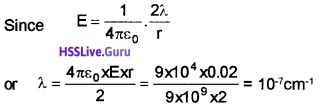### Plus Two Physics Electric Charges and Fields One Mark Questions and Answers

Question 1.
A point charge Q is place at the center of a cube of side, i the electric flux emerging the cube is.(d) $$\frac{q}{\varepsilon_{0}}$$

Question 2.
A hollowing insulated conduction sphere is given a positive charge of 10p. what will be the electric field at the center of the sphere if its radius is 2 meters?
(a) 20 μ Cm-2
(b) 5 Cm
(c) zero
(d) 8 Cm
(a) 20 μ Cm-2Question 3.
A charge Q μ C is placed at the center of a cube, the flux coming out from each face will be.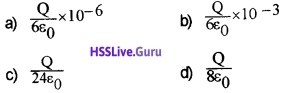(a) $$\frac{Q}{6 \varepsilon_{0}} \times 10^{-6}$$
Explanation: For complete cube Φ = $$\frac{Q}{\varepsilon_{0}} \times 10^{-6}$$
For each face Φ = $$\frac{1}{6}$$ $$\frac{Q}{\varepsilon_{0}} \times 10^{-6}$$

Question 4.
Pick the odd one out of the following based on electric field intensity.
(i) Point charge
(ii) Uniformly charged sphere
(iii) Uniformly charged cube
(iv) Uniformly charged sheet
(iv) Uniformly charged sheet (The electric field does not depend on distance)

Question 5.
“Electrostatic force is medium dependent that is why NaCI dissolves in water” comment on this statement.
Dielectric constant of water is 81. Hence the force between Na+ and cl reduces by 81 times.

Question 6.
Classify into true or false

1. In a charged conductor charges reside inside and out side the conductor
2. In a charged conductor net field is zero inside the conductor.

1. False
2. TrueQuestion 7.
Electrostatic force is a_____force
(i) Conservative
(ii) non conservative
(iii) medium independent
(iv) dissipative Conservative
(i) Conservative

### Plus Two Physics Electric Charges and Fields Two Mark Questions and Answers

Question 1.
It is safe to be inside a vehicle rather than outside, even when there is lightning and thunder. Comment on this.
Inside a spherical shell electrical field is zero. This is called electrostatic shielding. Hence it is safe to be inside a vehicle rather than outside.Question 2
There are two types of charges namely positive and negative.

1. List any two basic properties of electric charge.
2. Can a body have a charge of 0.8 × 1o-19 C? Which basic property of electric charge is the reason for your answer?

1. Quantization of electric charge. Conservation of electric charge.

2. No. According to quantisation of charge, total charge is Q = ne when ‘n’ is an intiger. Here
n = $$\frac{0.8 \times 10^{-19}}{1.6 \times 10^{-19}}$$ = 0.5 n is a fractional value.

### Plus Two Physics Electric Charges and Fields Three Mark Questions and Answers

Question 1.
A cubical iron block is given a charge of +40m C and placed in vacuum.

1. Give the direction of electric field at the centre of one face.
2. Calculate the net flux due to the charged cube through one face.
3. Will there be any change in the flux if it is placed underwater of dielectric constant 81. Justify.

1. Normally outwards from the surface.
Total flux = $$\frac{40 \times 10^{-3}}{8.85 \times 10^{-12}}$$ = 4.51 × 109Nm2C-1

2. Through one face = $$\frac{4.51 \times 10^{9}}{6}$$ = 0.75 × 109Nm2C-1

3. Electric flux decreases because flux depends on permittivity of medium.Question 2.
When a rubber sheet is rubbed with woolen carpet, the carpet is found to acquire a positive charge of 8 × 10-7C
1. In the above process charging is by

• conduction
• friction
• induction
• polarization

2. Among Rubber shoe and woolen carpet which one acquire both mass and charge during rubbing? Explain.
1. In the above process charging is by:

• Friction

2. Carpet is found to be positive body which means that electrons are lost from carpet to rubber shoe. Hence rubber shoe acquires charge and mass.

Question 3.
A glass rod rubbed with silk found to have acquired a positive charge 6.4 × 10-7C.
1. In the above process charging is by

• Induction
• Conduction
• Friction
• Electromagnetic induction

2. Regarding above process which statement is false

• glass rod loss some mass
• silk gain negative charge but no mass
• Silk gain charge arid mass
• Total charge of the silk glass system is zero

3. Will the above process obey law of quantization of electric charge? Clarify your answer by finding numbers of electrons transferred to silk.
1. In the above process charging is by:

• Friction

2. Regarding above process which statement is false:

• Silk gains charge and mass

3. yes
Number of electrons transferred to Silk,
n = $$\frac{q}{e}=\frac{6.4 \times 10^{-7}}{1.6 \times 10^{-19}}$$ = 4 × 1012Question 4.
In a NaCI molecule there is an attractive force between Na+ ion and Cl ion

1. Which law help us to find this attractive force? State the law.
2. State the law and write it’s mathematical form
3. Why NaCI molecule is easily soluble in water? [Hint;- Dielectric constant of water is 81]

1. Coulombs law.

2. The force between two changes is directly proportional to product of their charges and inversely proportional to square of the distance between them. Force on q1 due q2.

3. Force between two charges depends on the medium in between them.
i.e. F = $$\frac{1}{4 \pi \varepsilon_{0} k} \frac{q_{1} q_{2}}{r^{2}}$$
For water molecule K=81. Hence the force between two charge in water decreases by 81 times. As result NaCl molecule is easily soluble in water.

Question 5.
There are two types of charges namely positive and negative.
1. List any two basic properties of electric charge. (1)

2. Can a body have a charge of 0.8 × 10-19 C? Which basic property of electric charge is the reason for your answer? (1)

3. Name the basic property of electric charge that you can see in the equation. (1)
Rn86 → P084 + He2

1. Quantization of electric charge. Conservation of electric charge.

2. No. According to quantisation of charge, total charge is Q = ne when ‘n’ is an intiger. Here
n = $$\frac{0.8 \times 10^{-19}}{1.6 \times 10^{-19}}$$ = 0.5 n is a fractional value.Question 6.
The rectangular block shown in the figure attracts the flowing water.Identify the rectangular block as a magnet or electrically charged body. Justify your answer.

• Electrically charged body.
• Charged body attracts water flow.

Question 7.
Figure represent an infinite sheet of charge of surface charge density σ. You have to find electric field intensity at P due to this infinite charge.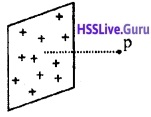1. Can you apply Coulomb’s inverse square law to find electric field at P?
2. Find electric field intensity at P due to this sheet

1. No.

2. Field due to A uniformly charged infinite plane sheet:Consider an infinite thin plane sheet of change of density σ.
To find electric field at a point P (at a distance ‘r’ from sheet), imagine a Gaussian surface in the form of cylinder having area of cross section ‘ds’.
According to Gauss’s law we can write,But electric field passes only through end
surfaces ,so we get ∫ ds = 2dsE is directed away from the charged sheet, if σ is positive and directed towards the sheet if σ is negative.

### Plus Two Physics Electric Charges and Fields Four Mark Questions and Answers

Question 1.
Two charges +3µC and -3µC are separated by a very small distance of 5mm.
1. What is the name of the above arrangement?

2. If the above arrangement is placed in a uniform electric field of intensity 3 × 10-5 N/C with its axis perpendicular to the field direction. What is the torque acting on it?

3. If the arrangement is placed in a non uniform electric field, what happens?

1. Electric dipole.

2. τ = P × E
= PE (θ = 90°) = q2aE
= 3 × 10-6 × 5 × 10-3 × 3 × 10-5 Nm(∴ 2a = 5 × 10-3)

3. The dipole undergoes for both translation and rotational motion.Question 2.
NaCI when placed in a uniform electric field 20VM-1, both Na+ and Cl ions experience a force (average distance between Na+ and Cl is 1.3A°).

1. In what way the NaCI will orient in the electric field.
2. Arrive an expression for torque experienced by the dipole.
3. Calculate the maximum torque experience by the NaCl molecule in the electric field.

1. ‘Na’ is orienting towards -ve side and cl ions towards +ve side.

2.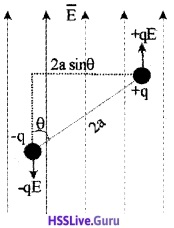Consider an electric dipole of dipole moment P = 2aq kept in a uniform external electric field, inclined at an angle θ to the field direction.
torque = any one force × perpendicular distance τ = qE × 2 a sin θ
Since P = 2aq
τ = p E sin θ
Vectorialy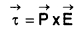3. τ = q2a.E
τ = 1.6 × 10-19 × 1.3 × 10-10 × 20 Nm
= 41.6 × 10-29 Nm.Question 3.
A metal sphere of radius R carrying q (+ve) charges is shown in the figure.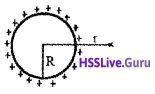1. Draw the electric lines of force related to this metal sphere.

2. Derive the strength of electric field at a distance ‘r’ from the centre of sphere.

3. “A sphere of radius 1cm can hold a charge of 1 coulomb” Comment on the statement.
[Hint:- The dielectric strength of air is 3 × 106 v/m]
1.2. Field due to A uniformly charged thin spherical shell:
Consider a uniformly changed hollow spherical conductor of radius R. Let ‘q’ be the total charge on the surface.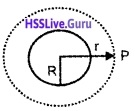To find the electric field at P (at a distance r from the centre), we imagine a Gaussian spherical surface having radius r.
Then, according to Gauss’s theorem we can write,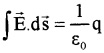The electric field is constant, at a distance ‘r’. So we can write,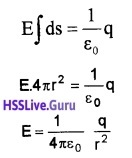Case -1: Electric field inside the shell is zero.
Case – II: At the surface of shell r = R
∴ E = $$\frac{1}{4 \pi \varepsilon_{0}} \frac{q}{R^{2}}$$

3. The Electric field intensity due to Ic,E = 9 × 1013v/m.
This Electric field is higher than the dielectric strength of air (3 × 106v/m). Hence the charge will leak through air.Question 4.
Two metal plates A and B are connected to cell of emf 2V is shown in the figure1. Redraw the diagram and draw lines of forces to represent electric field.
2. Calculate the value of electric field between A and B.
3. A charged particle starting from rest moves in the opposite direction of electric field, is it an electron or proton?
4. Calculate acceleration of above particle.

1. Diagram and draw lines of forces to represent electric field:2. E = $$\frac{v}{d}=\frac{2}{1 \times 10^{-3}}$$ = 2 × 103 v/m.

3. Electron. Electron flows from lower potential to higher potential ie. flows from -ve terminal to +ve terminal.

4. F = eE , ma = eE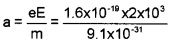a = 3.5 × 1014 m/sec2.

Question 5.
Two closed surface S1 and S2 enclose two charges q1 and q2 as shown in the figure.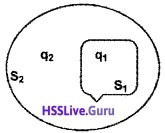1. State the law in electrostatic that relates the electric flux passing through the surface with the charge enclosed. (1)
2. If q1 = +6µC and q2 = -4µC find the ratio of the flux passing through surfaces S1 and S2. (2)
3. Let the surface S2 expands to double its area while S1 remains as such. What will happen to the above ratio? (1)

1. The net flux of electric field passing through a closed surface is equal to 1/ε0 times the charge enclosed by the surface.

2. By Gauss’ theorem flux passing through a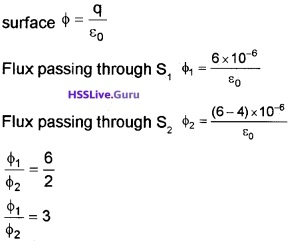3. Remains the same (because electric flux is independent of the size and shape of the enclosed surface).Question 6.
A spherical shell of radius R is uniformly charged to a surface charge density σ.

1. State the theorem which can be used to find the electric field outside the shell.
2. Using the theorem arrive at an expression for electric field at a distance r from the centre of the spherical shell.
3. It is safe to be inside a vehicle rather than outside, even when there is lightning and thunder. Comment on this. (HSES.Q.P)

1. Gauss’s theorem states that the total electric flux over a closed surface is 1/ε0 times the total charge enclosed by the surface.

2. Field due to A uniformly charged thin spherical shell:
Consider a uniformly changed hollow spherical conductor of radius R. Let ‘q’ be the total charge on the surface.To find the electric field at P (at a distance r from the centre), we imagine a Gaussian spherical surface having radius r.
Then, according to Gauss’s theorem we can write,The electric field is constant, at a distance ‘r’. So we can write,Case -1: Electric field inside the shell is zero.
Case – II: At the surface of shell r = R
∴ E = $$\frac{1}{4 \pi \varepsilon_{0}} \frac{q}{R^{2}}$$.

3. Inside a spherical shell electrical field is zero. This is called electrostatic shielding. Hence it is safe to be inside a vehicle rather than outside.Question 7.
q1, q2, and q3 are three point charges located in free space

1. What is the force between q1 and q2, if q3 is absent?
2. What is the force between q1 and q2 if q3 is present?
3. State the principle used to find total force on q1 and calculate total force F1 on q1
4. Draw the vector diagram representing individual forces and total force on q1.

1.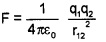2.3. Total force on q1 can be found using super position principle ie.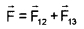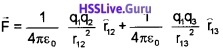Where r12 is the distance between q1 and q2 and r13 is the force between q1 and q3Question 8.
Variation of field with distance of a charged conducting shell is given below. R is the radius of the sphere
1. field is maximum at

• centre of the sphere
• at r<R
• at r>R
• at r = R (surface)

2. From the graph find value of field at centre. Give on practical application of this result

3. Draw the potential -distance graph in the above case1. at r = R

2. E = 0

• It is safe to be sit inside car during lightning
• Sensitive electrical instruments can be pro-tected from external electric field by placing inside a metal box.

3.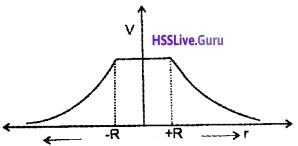### Plus Two Physics Electric Charges and Fields Five Mark Questions and Answers

Question 1.
Two-point charges q1 and q2 are separated by a distance ‘r’ in space
1. What happens to the force between the charges;

• When the magnitude of the point charges increases
• When the distance of separation decreases

2. Three-point charges +2µC each are placed at the corners of an equilateral triangle of side 1 m. Find the magnitude of the force between charge q1 and q1 [F12] and q1 and q3 [F13].

3. Draw $$\overrightarrow{\mathrm{F}}_{12}$$ and $$\overrightarrow{\mathrm{F}}_{13}$$ at A and find the total force acting on q1.
1. The force between the charges:

• Force increases
• Force decreases
$$F=\frac{1}{4 \pi \varepsilon_{0}} \frac{q_{1} \times q_{2}}{r^{2}}$$

2. Force between q1 and q2= 36 × 10-3 N.

3.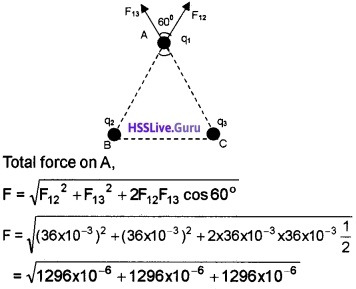= 62.35 × 10-6 N.Question 2.
Match the following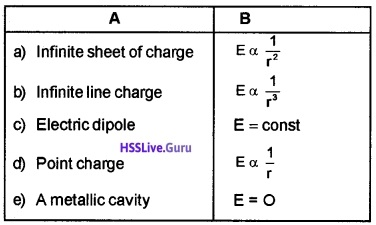(a) E = constant
(b) E ∝ 1/r
(c) E ∝ 1/r3
(d) E ∝ 1/r2
(e) E = O

Question 3.
An electric dipole is placed in a uniform electric field . of Intensity E as shown in the figure.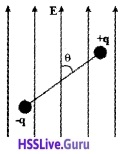1. What are the forces on +q and -q?
2. What is the net force on the system?
3. Copy the diagram, mark the forces and derive an expression for torque.
4. If the dipole is placed in a non uniform electric field what nature of motion does it show?

1. Force on +q, F+ = +qE (along the direction of electric field)
Force on -q, F = -qE (Opposite to the direction of electronic field)

2. Net force on the system is zero.

3.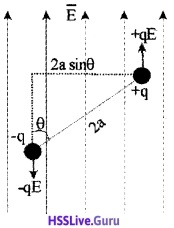Consider an electric dipole of dipole moment P = 2aq kept in a uniform external electric field, inclined at an angle θ to the field direction.
torque = any one force × perpendicular distance τ = qE × 2 a sin θ
Since P = 2aq
τ = p E sin θ
Vectorialy4. In nonuniform electric field, total force is not zero. Hence the dipole undergoes for both translational and rotational motion.Question 4.
In electrostatics, Electric charge is a feature of particles like protons, electrons, etc. that decides the
force of interaction among them.
1. Write the name of the law that is used to measure the above force of interaction.

2. Express the above law in vector form.

3. Two charged spheres when placed in air attract with a force F. Keeping the distance between the charges constant, the spheres are immersed in a liquid of relative permittivity K. Then the spheres will.

• attract with a force KF
• repel with a force KF
• attract with a force F/K
• repel with a force F/K

4. Two small aluminum spheres are separated by 80cm. How many electrons are to be transferred from one sphere to the other so that they attract with a force of 104N.
1. Coulomb’s law in electrostatics

2.3. Attract with a force F/K

4. If n electrons are transferred, charge on each sphere Q = ne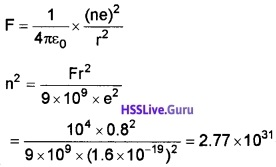n = 5.27 × 1015

Question 5.
Intensity of electric field is a vector quantity.
1. Define intensity of electric field at a point.

2. Two small spheres A and B carrying charges 2µC and 6µC respectively are separated by a fixed distance. Intensity of electric field at the location of B due to A is E. Intensity of electric field at the location of A due B is

• E
• 3E
• 6E
• 12E

3. Two-point charges +4nC and +5nC are placed at x = 0.2m and x = 0.3m respectively along the x-axis. Find the magnitude and direction of intensity of electric field at the origin.
1. Intensity of electric field at point is the electrostatic force experienced by unit positive charge placed at that point.

2. 3E

3.Intensity of electric field at the origin due to the negative chargeE(-) = 500NC-1 along -ve x axis
Intensity of electric field at the origin due to the positive charge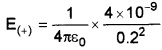E(+) = 900NC-1 along -ve x axis
Net intensity of electric field at the origin
E = E(+) + E(-) = 500 + 900 = 1400 NC-1 along -ve x axis.Question 6.
You find a sealed box on your door step. You suspect that the box contain charged metal spheres packed in insulating material.

1. Which law explains relation between charge of spheres and electric flux.
2. State the law.
3. Give a mathematical proof for this law.
4. Using this law, can you estimate the total charge inside the box without opening the box?

1. Gauss’s theorem

2. Gauss’s theorem states that electric flux over a closed surface is 1/ε0 times the total charge enclosed by the surface.
Gauss’s theorem may be expressed as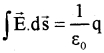3. Gauss’S Law:
Gauss’s theorem states that the total electric flux over a closed surface is 1/ε0 times the total charge enclosed by the surface.
Gauss’s theorem may be expressedProof: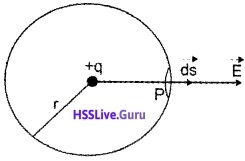Consider a charge +q .which is kept inside a sphere of radius ‘r’.
The flux at ‘P’ can be written as,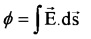But electric field at P, E =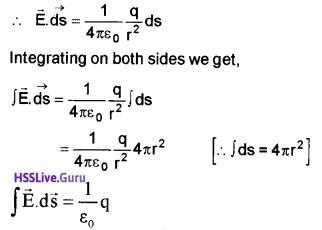Important points regarding Gauss’s law:

• Gauss’s law is true for any closed surface.
• Total charge enclosed by the surface must be added (algebraically). The charge may be located anywhere inside the surface.
• The surface that we choose for the application of Gauss’s law is called the Gaussian surface.
• Gauss’s law is used to find electric field due to system of charges having some symmetry.

4.Question 7.

• Write an expression to find electric flux passing through small element ds
• Which law helps to find total flux passing through a closed surface? State the law.
• Using this law, derive an expression for electric field due to thin uniformly charged shell.
• How can we protect delicate instruments from external electric field?1.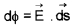2. Gauss’s law:
Guass’s law states that total flux over a closed surface is 1/s0 times net charge enclosed by the surface.

3. Field due to A uniformly charged infinite plane sheet: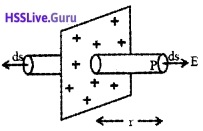Consider an infinite thin plane sheet of change of density σ.
To find electric field at a point P (at a distance ‘r’ from sheet), imagine a Gaussian surface in the form of cylinder having area of cross section ‘ds’.
According to Gauss’s law we can write,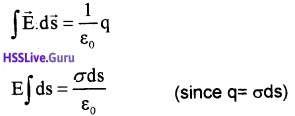But electric field passes only through end
surfaces ,so we get ∫ ds = 2ds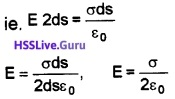E is directed away from the charged sheet, if σ is positive and directed towards the sheet if σ is negative.

4. Delicate instruments can be protected from ex-ternal electric field by keeping it in a metal cavity.